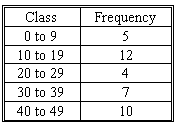### CFA Practice Question

There are 985 practice questions for this topic.

### CFA Practice Question

Use the following frequency distribution:The median of this distribution is ______.
A. 24.5
B. 25.5
C. 26.5
Explanation: The median data can be calculated by:

median = L + (( n / 2 - CF) * I ) / F

n is the number of items in the sample.
L represents the lower boundary of the class containing the median.
CF is the cumulative frequencies of all the classes prior to the one containing the median.
F represents the frequency in the median class.
I is the interval size of the class containing the median.

In this case, n = 38, L = 20, CF = 17, F = 4, I = 9
Therefore:
median = 20 + ((38/2 - 17) * 9) / 4 = 20 + ((19 - 17) * 9) / 4 = 20 + (2 * 9) / 4 = 20 + 18 / 4 = 20 + 4.5 = 24.5

User Comment
chamad what if we take the middle point of the median interval (29+20):2=24.5? does it always work?
capitalpirate no, worked here because median in 19th and 20th term, which are the two middle in the interval... if n>4, then it would be different...
kellyyang can anyone by explaining this question step by step pleased? I could not figure out the solution!
thanks!
ksnider that formula is too complicated to remember and it might not apply to every single question, i'd rather just count it out and prove which is the median by hand
endurance actually iy is quite - just think intuively.
The median is in the middle some where, which means that L starts at 20.

Take the total numbers 38 and divide it by 2 (n/2).

Remove the cumulative distibution -> 20+(38/2-17)

Now lets move to inside the class and find the interval -> class size is 9 and we've got 4 numbers -> 9/4.

So lets put the whole thing together -> median=20+(38/2-17)*(9/4)=24,5
Shaan23 Im a stats guy. This is how you do it. There's 38 observations therefor the 19.5th observation is the median # we're looking for. The 19.5th number is in the range of (20,29). We can see that there is a frequency of 4 in that range so split it up into four numbers corresponding to each frequency (20, 23, 26, 29). The 19th number corresponds to 23 and the 20th number to 26. Average those two numbers and you get 24.5
renataa Thanks Shaan23, your logic is easier than the furmula
wenhu the fomula is more logical